# Tag like an Egyptian

Ajay Puvvala is my friend and colleague at the MathWorks. He also led the development of the TestTags feature we spoke about last post.

Ajay, as per usual, made a great comment after last week's post which I wanted to highlight here. It had to do with the test pyramid: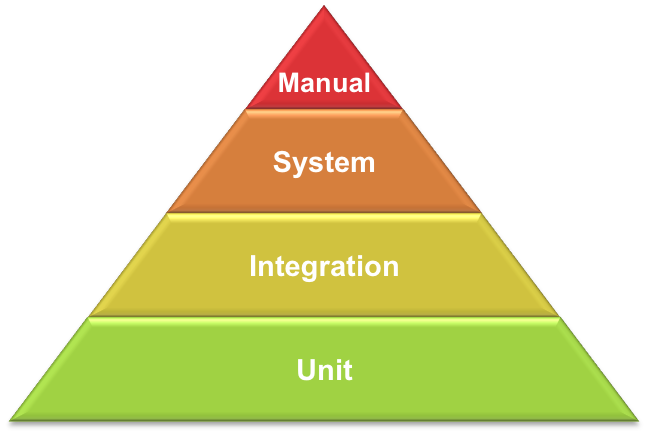You know the test pyramid (another)? I am sure we will have more posts discussing it in the future, but until then the fundamental principle is that you want to form a solid foundation of unit tests followed by fewer integration tests and even fewer system tests. Manual regression tests (not to be confused with exploratory and/or user testing) should be kept to an absolute minimum, although we shouldn't ignore them when needed. The rationale here is that the farther you go up in the testing pyramid the more expensive these tests become. They are harder to write, to maintain, to run, and to make robust. They are needed, but we should opt for the pyramid as opposed to the ice cream cone.

Anyway, Ajay mentioned that a test suite using tags such as the Small/Medium/Large tags discussed last post can help us get a sense of the health of our pyramid or ice cream cone. Yes I am a realist, I know that many of us have cones instead of pyramids. How do we get a sense of where we are?

To see this I will create a few tests that leverage test parameterization to quickly create a large test array.

classdef PyramidSuite < matlab.unittest.TestCase
% This test class contains a nice pyramid distribution of unit tests to
% system tests.

properties(TestParameter)
% To create a representative suite, create 3 parameters and implement test
% methods and use different subsets of these parameters in the test
% methods. Since they are combined exhaustively, the more parameters a test
% uses the more test elements will get created.
param1 = num2cell(1:20);
param2 = num2cell(1:5);
param3 = num2cell(1:3);
end

methods(Test, TestTags={'Small'})
function smallTest(testCase, param1, param2, param3)
end
end

methods(Test, TestTags={'Medium'})
function mediumTest(testCase, param1, param2)
end
end

methods(Test, TestTags={'Large'})
function largeTest(testCase, param1)
end
end

end



Here I have created a nicely balanced test suite with a healthy use of small tests and lower use of larger tests in the suite. How can I be sure of this? By looking at the test tags.

import matlab.unittest.TestSuite;
pyramidSuite = TestSuite.fromClass(?PyramidSuite);

pyramidSizes = categorical([pyramidSuite.Tags]);
pie(pyramidSizes, [1 1 1]);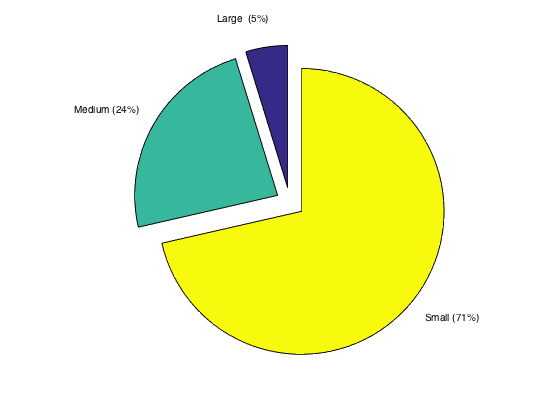Look at that! Super simple to get a high level view of your test bed. Note this does assume that each test is tagged with one and only one of the Small/Medium/Large tags we are operating with here.

However this doesn't look like a pyramid, it looks like a pie! Alright, just for fun let's make our own test pyramid with our test tag data. We'll start by getting the tag counts, sorting them so we can build our pyramid correctly, and assign colors to reflect our preference for smaller, less expensive tests.

[counts, sizes] = histcounts(pyramidSizes);

% Sort the values to allow creation of the pyramid
[counts, index] = sort(counts);
sizes = sizes(index);

% Assign:
% small tests: green
% medium tests: yellow
% large tests: red
colors{strcmp(sizes,'Small')} = 'g';
colors{strcmp(sizes,'Medium')} = 'y';
colors{strcmp(sizes,'Large')} = 'r';


Great, now we can calculate a few values for the sizes of our triangles required to create a stacked pyramid where the area of each stack matches the percentage of the test suite matching the category. I'll leave the calculation here as an exercise for the reader, but the constraints at play are that the area of the largest containing triangle is 1, it's base and height are both chosen to be $\sqrt{2}$, and the areas of each section match the percentage of corresponding categories in the suite.

total = sum(counts);
percentMiddle = counts(2)/total;
percentTop = counts(1)/total;

heightBottom = sqrt(2);
heightMiddle = sqrt(2*percentMiddle+percentTop);
heightTop = sqrt(2*percentTop);

baseBottom = sqrt(2);
baseMiddle = (heightMiddle/heightBottom)*baseBottom;
baseTop = (heightTop/heightBottom)*baseBottom;


Now create the endpoints of each trapezoid and the top triangle using these dimensions. Place the endpoints into an Nx2 array corresponding to (x,y) coordinates.

%Bottom section
bottom(1,:) = [0 0];
bottom(2,:) = [(baseBottom-baseMiddle)/2, heightBottom-heightMiddle];
bottom(3,:) = [bottom(2,1) + baseMiddle, bottom(2,2)];
bottom(4,:) = [baseBottom, 0];

% Middle section
middle(1,:) = bottom(2,:);
middle(2,:) = [middle(1,1) + (baseMiddle-baseTop)/2, heightBottom-heightTop];
middle(3,:) = [middle(2,1) + baseTop, middle(2,2)];
middle(4,:) = bottom(3,:);

% Top section
top(1,:) = middle(2,:);
top(2,:) = [baseBottom/2, heightBottom];
top(3,:) = middle(3,:);


...and create the pyramid using fill and add labels at the centroid of each region.

fill(bottom(:,1), bottom(:,2), colors{3}, ...
middle(:,1), middle(:,2), colors{2}, ...
top(:,1), top(:,2), colors{1});
axis off;

findCentroid = @(points) sum(points,1)/length(points);

topCentroid = findCentroid(top);
text(topCentroid(1), topCentroid(2), sizes{1}, ...
'HorizontalAlignment', 'center', 'FontSize', 14,'FontWeight', 'bold');

middleCentroid = findCentroid(middle);
text(middleCentroid(1), middleCentroid(2), sizes{2}, ...
'HorizontalAlignment', 'center', 'FontSize', 14,'FontWeight', 'bold');

bottomCentroid = findCentroid(bottom);
text(bottomCentroid(1), bottomCentroid(2), sizes{3}, ...
'HorizontalAlignment', 'center', 'FontSize', 14,'FontWeight', 'bold');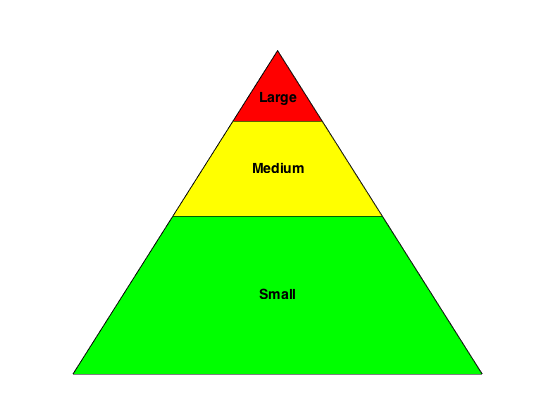There ya have it, a true testing pyramid built from the data found in the test tags. To convince ourselves this is working lets put it in a function and try it out on a different suite. Here is the function:

function handles = testPyramid(testSizes)
[counts, sizes] = histcounts(testSizes);

% Sort the values to allow creation of the pyramid
[counts, index] = sort(counts);
sizes = sizes(index);

% Assign:
% small tests: green
% medium tests: yellow
% large tests: red
colors{strcmp(sizes,'Small')} = 'g';
colors{strcmp(sizes,'Medium')} = 'y';
colors{strcmp(sizes,'Large')} = 'r';

total = sum(counts);
percentMiddle = counts(2)/total;
percentTop = counts(1)/total;

heightBottom = sqrt(2);
heightMiddle = sqrt(2*percentMiddle+percentTop);
heightTop = sqrt(2*percentTop);

baseBottom = sqrt(2);
baseMiddle = (heightMiddle/heightBottom)*baseBottom;
baseTop = (heightTop/heightBottom)*baseBottom;

% Bottom section
bottom(1,:) = [0 0];
bottom(2,:) = [(baseBottom-baseMiddle)/2, heightBottom-heightMiddle];
bottom(3,:) = [bottom(2,1) + baseMiddle, bottom(2,2)];
bottom(4,:) = [baseBottom, 0];

% Middle section
middle(1,:) = bottom(2,:);
middle(2,:) = [middle(1,1) + (baseMiddle-baseTop)/2, heightBottom-heightTop];
middle(3,:) = [middle(2,1) + baseTop, middle(2,2)];
middle(4,:) = bottom(3,:);

% Top section
top(1,:) = middle(2,:);
top(2,:) = [baseBottom/2, heightBottom];
top(3,:) = middle(3,:);

handles = fill(bottom(:,1), bottom(:,2), colors{3}, ...
middle(:,1), middle(:,2), colors{2}, ...
top(:,1), top(:,2), colors{1});
axis off;

handles(end+1) = labelSections(top, sizes{1});
handles(end+1) = labelSections(middle, sizes{2});
handles(end+1) = labelSections(bottom, sizes{3});

function textHandle = labelSections(points, label)
centroid = sum(points,1)/length(points);
textHandle = text(centroid(1), centroid(2), label, ...
'HorizontalAlignment', 'center', 'FontSize', 14,'FontWeight', 'bold');



Create the suite using the same parameterized testing tactic, but this time try it on a poorly implemented suite that relies too much on 'Large' tests. I want to build the ice cream cone:

classdef IceCreamConeSuite < matlab.unittest.TestCase
% This test class clearly relies on a bit too many large tests and not
% enough small tests.

properties(TestParameter)
% Tweak the distribution of our parameters a bit from
% the PyramidSuite to create different percentages
param1 = num2cell(1:20);
param2 = num2cell(1:2);
param3 = num2cell(1:2);
end

methods(Test, TestTags={'Small'})
function smallTest(testCase, param1)
end
end

methods(Test, TestTags={'Medium'})
function mediumTest(testCase, param1, param2)
end
end

methods(Test, TestTags={'Large'})
function largeTest(testCase, param1, param2, param3)
end
end

end


iceCreamConeSuite = TestSuite.fromClass(?IceCreamConeSuite);

iceCreamConeSizes = categorical([iceCreamConeSuite.Tags]);

handles = testPyramid(iceCreamConeSizes);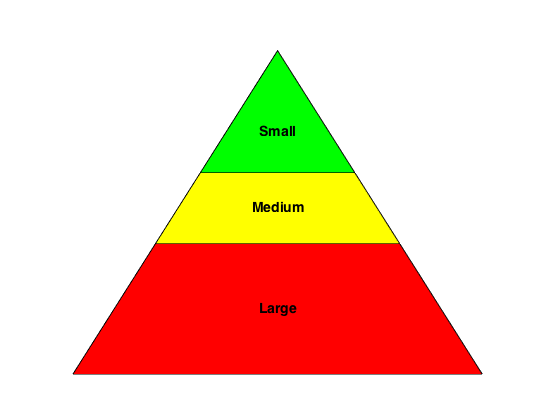Looks correct, we now can simply rotate it to create the ice cream cone.

rotate(handles, [0 0 1], 180);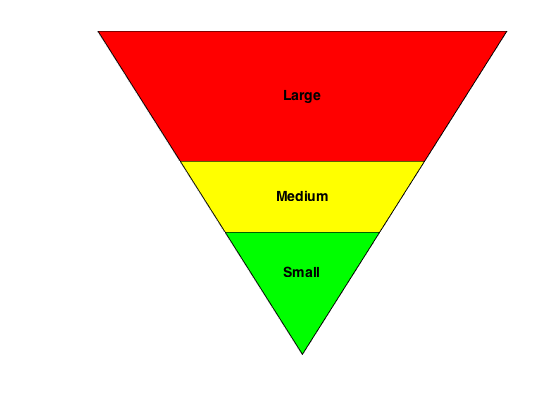Do you strive for the test pyramid? Have you found any other methods for introspecting into your test bed to see how you are doing?

Published with MATLAB® R2015b

|